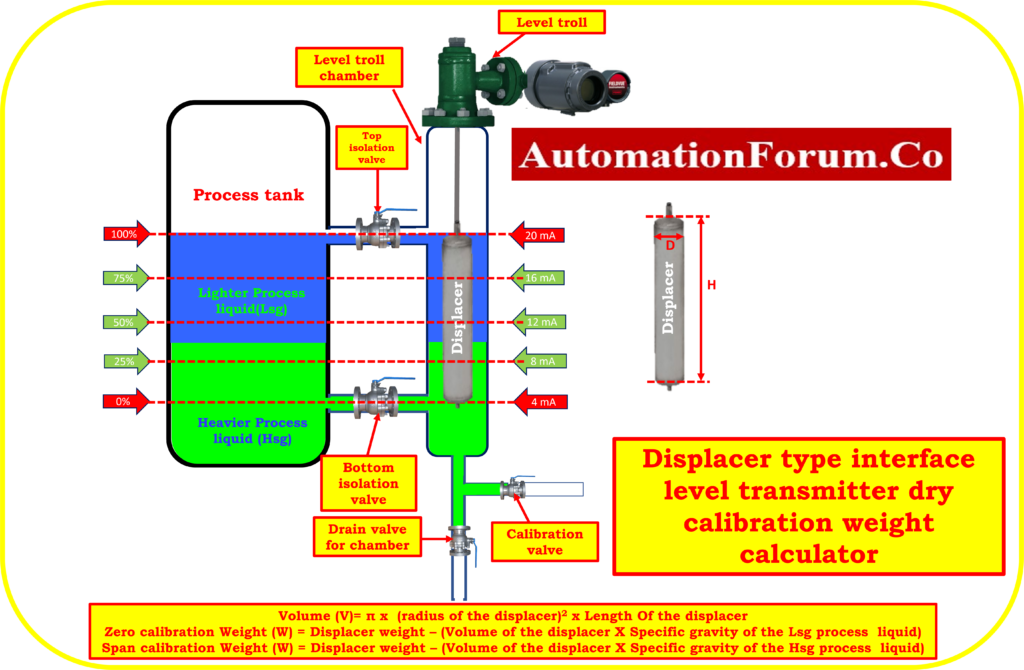# Displacer type interface level transmitter dry calibration weight calculatorThe Displacer interface level transmitter dry calibration weight calculator is a valuable tool used in industrial settings for the calibration of displacer interface level transmitters without the need for process liquid.

• The Displacer type interface level transmitter dry calibration weight calculator is significant as it ensures accurate calibration of the transmitter, leading to reliable interface level measurements.
• It saves time and reduces costs by providing precise weight values for calibration, eliminating trial and error.
• Proper calibration maintains consistency and reliability, optimizing industrial processes and ensuring safety and environmental compliance.
• Proper calibration using the weight calculator ensures consistency and reliability of the interface level transmitter over time.

## Formula for the volume of the displacer

Volume of the displacer   V = πr2h

Where,

r = Radius of the displacer.

h = Length of the displacer.

D = Diameter of the displacer

## Formula for calculating the displacer weight loss due to process liquid

Displacer weight loss due to process liquid =Volume of the displacer X Specific gravity of the process liquid

## Formula for zero apparent weight calibration

Weight for zero calibration = Displacer Actual Weight(W) – (Displacer Actual Volume X specific gravity of the Lsg liquid)

Where

Lsg = Specific gravity lighter process liquid

W = Actual weight of the displacer

## Formula for span apparent weight calibration

Weight for Span calibration = Displacer Actual Weight(W) – (Displacer Actual Volume X specific gravity of the Hsg liquid)

Where

Hsg = Specific gravity heavier process liquid

W = Actual weight of the displacer

## Example calculation

Displacer and process liquid characteristics:

W = Displacer weight is 2400 Gram

D = Displacer diameter is 7.0 Centimeter

H = Length of Displacer is 32.42 Centimeter

Lsg = Specific gravity of lighter process liquid is 0.7

Hsg = Specific gravity of Heavier process liquid is 1.0

V = Volume of displacer.

So,

Radius of the displacer r = 7.0/2 = 3.5 centimeters

Displacer Actual volume V = πr2h

Volume V = 3.14 × r² × h

= 3.14 × 3.5 × 3.5 × 32.42 = 1247.04 cubic centimeter

## Zero calibration weight value calculation (0%)

Displacer weight loss due to lighter process liquid = Displacer Actual Volume X Specific gravity of the Lsg liquid

Weight for zero calibration = Displacer Actual Weight (W) – (Displacer Actual Volume X Specific gravity of the Lsg liquid

Weight for zero calibration = 2400 – (1247 X 0.7)

= 2400 – 872.9

Weight for zero calibration =1527.1 grams

## Span calibration weight value calculation (100%)

Displacer weight loss due to heavier process liquid = Displacer Actual Volume X Specific gravity of the Hsg liquid

Weight for Span calibration = Displacer Actual Weight (W) – (Displacer Actual Volume X specific gravity of the Hsg liquid)

Weight for Span calibration = 2400 – (1247 X 1.0)

= 2400 – 1247

Weight for Span calibration =1153 grams

## Weight for each 25% calibration check of linearity

Actual span weight is calculated by subtracting the weight lost by the displacer due to the heavier process liquid (Hsg) and the lighter specific gravity liquid (Lsg).

Actual span weight = 1247 – 872.9

Actual span weight = 374.1

For each 25% = Actual span weight/4

= 374.1/4

For each 25% = 93.525 grams